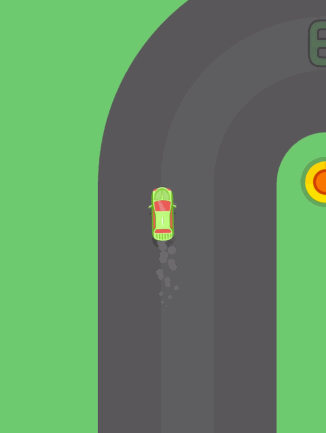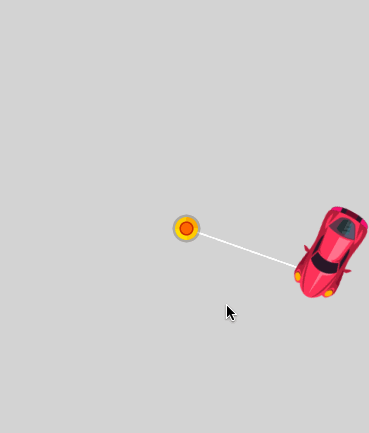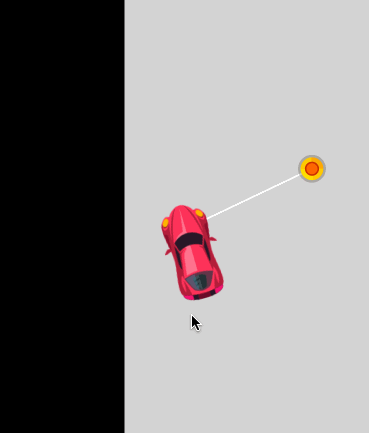# CocosCreator的MotionStreak组件实现轮胎印

### 分析### 实现Creator的拖尾正好就是渐变的效果，但那适用于短时间的情况，拖尾停留的时间比较长，所以需要对图片做一点处理，做成渐变的图，可以Demo中查看。

```this.carHead.rotation = cc.radiansToDegrees(cc.pAngleSigned(cc.pSub(this.carHead.position, this.carTail.position), cc.p(0, 1)));

```let speedX = 2000 * Math.sin(this.carHead.rotation * Math.PI / 180);
let speedY = 2000 * Math.cos(this.carHead.rotation * Math.PI / 180);

```this.tireStreak_left.position = cc.pSub(this.posTireLeft.convertToWorldSpace(cc.v2(0, 0)), cc.v2(cc.winSize.width / 2, cc.winSize.height / 2));
this.tireStreak_right.position = cc.pSub(this.posTireRight.convertToWorldSpace(cc.v2(0, 0)), cc.v2(cc.winSize.width / 2, cc.winSize.height / 2));```

`this.cameraNode.position = this.carHead.position;`